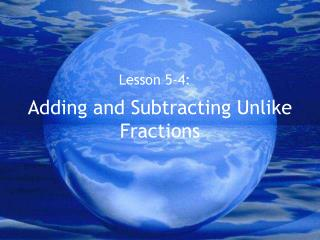DownloadDownload Presentation# Adding and Subtracting Unlike Fractions

Télécharger la présentation## Adding and Subtracting Unlike Fractions

- - - - - - - - - - - - - - - - - - - - - - - - - - - E N D - - - - - - - - - - - - - - - - - - - - - - - - - - -
##### Presentation Transcript

1. Adding and Subtracting Unlike Fractions Lesson 5-4:

2. Rewrite the problem with equivalent fractions • List the multiples of both denominators. • Find the least common multiple (LCM). • Write new fractions with the LCM as the new denominator. • Find the factor you multiply by to get from your original denominator to your new denominator. • Use that same factor, and multiply it by your original numerator to get a new numerator.

3. WHAT DOES THAT MEAN? Let’s illustrate the steps with an example. 3 1 + 4 6

4. 3 1 + 4 6 9 2 x 3 x 2 + 12 12 11 Multiples of 4: 4, 8, 12, 16, 20 12 Multiples of 6: 6, 12, 18, 24, 30

5. Example 2 9 2 10, 20, 30, 40, 50 5, 10, 15, 20, 25 5 10 5 x 1 x 2 9 4 = 10 10 10 1 = 2

6. Practice Time!P266 # 12-15